Question

# You wish to make a 0.132 M nitric acid solution from a stock solution of 6.00...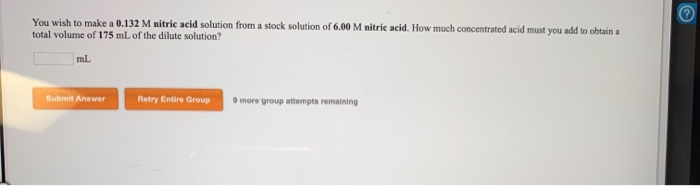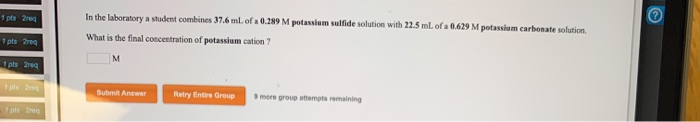You wish to make a 0.132 M nitric acid solution from a stock solution of 6.00 M nitric acid. How much concentrated acid must you add to obtain total volume of 175 mL of the dilute solution? Submit Answer Retry Entire Group more group attempts remaining
1 pts are In the laboratory a student combines 37.6 ml of a 0.289 M potassium sulfide solution with 22.5 ml of a 0.629 M potassium carbonate solution What is the final concentration of potassium cation ? 1 pts M pts 2009 pere Submit Answer Retry Entre Group more group attempts remaining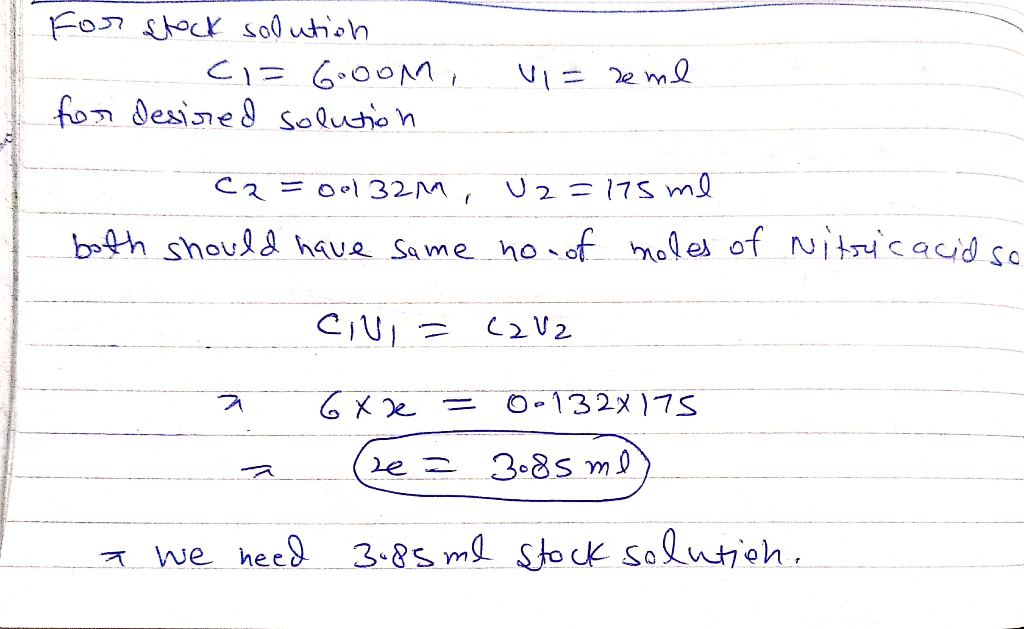#### Earn Coins

Coins can be redeemed for fabulous gifts.

Similar Homework Help Questions
• ### You wish to make a 0.257 M nitric acid solution from a stock solution of 3.00...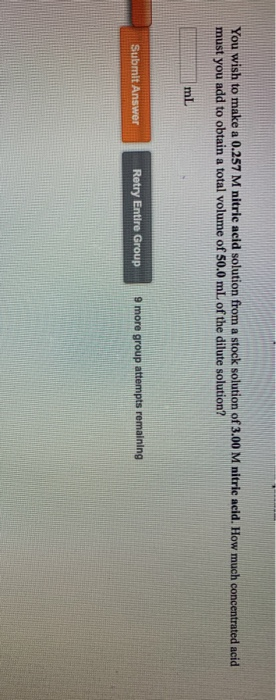You wish to make a 0.257 M nitric acid solution from a stock solution of 3.00 M nitric acid. How much concentrated acid must you add to obtain a total volume of 50.0 mL of the dilute solution? Submit Answer Retry Entire Group 9 more group attempts remaining

• ### You wish to make a 0.135 M nitric acid solution from a stock solution of 3.00...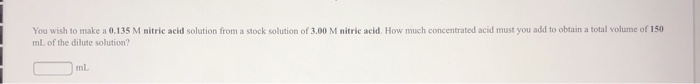You wish to make a 0.135 M nitric acid solution from a stock solution of 3.00 M nitric acid. How much concentrated acid must you add to obtain a total volume of 150 mL of the dilute solution? ml

• ### You wish to make a 0.252 M nitric acid solution from a stock solution of 12.0...

You wish to make a 0.252 M nitric acid solution from a stock solution of 12.0 M nitric acid. How much-concentrated acid must you add to obtain a total volume of 175 mL of the dilute solution?

• ### You wish to make a 0.249 M hydrobromic acid solution from a stock solution of 12.0...

You wish to make a 0.249 M hydrobromic acid solution from a stock solution of 12.0 M hydrobromic acid. How much concentrated acid must you add to obtain a total volume of 50.0 mL of the dilute solution? __mL You wish to make a 0.112 M nitric acid solution from a stock solution of 12.0 M nitric acid. How much concentrated acid must you add to obtain a total volume of 150 mL of the dilute solution? __mL In the...

• ### How many milliliters of 7.22 M nitric acid solution should be used to prepare 3.00 L...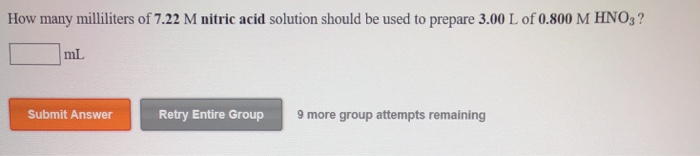How many milliliters of 7.22 M nitric acid solution should be used to prepare 3.00 L of 0.800 M HNO3? mL Submit Answer Retry Entire Group 9 more group attempts remaining

• ### A 23.0 mL sample of a 0.497 M aqueous acetic acid solution is titrated with a...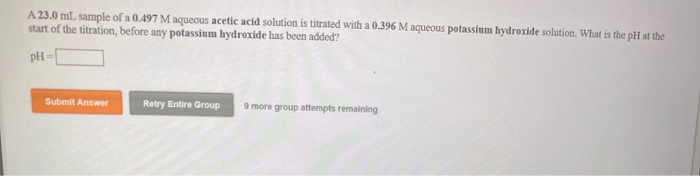A 23.0 mL sample of a 0.497 M aqueous acetic acid solution is titrated with a 0.396 M aqueous potassium hydroxide solution. What is the pH at the start of the titration, before any potassium hydroxide has been added? PH Submit Answer Retry Entire Group 9 more group attempts remaining What is the pH at the equivalence point in the titration of a 25.5 mL sample of a 0.411 M aqueous acetic acid solution with a 0.352 M aqueous barium...

• ### 1b. You wish to make a 0.395 M perchloric acid solution from a stock solution of...

1b. You wish to make a 0.395 M perchloric acid solution from a stock solution of 3.00 M perchloric acid. How much concentrated acid must you add to obtain a total volume of 75.0 mL of the dilute solution? 1c. In the laboratory you dilute 4.36 mL of a concentrated 12.0 M perchloric acid solution to a total volume of 50.0 mL. What is the concentration of the dilute solution?

• ### A 21.2 ml sample of 0.217 M ethylamine, CH_NH, is titrated with 0.287 M nitric acid....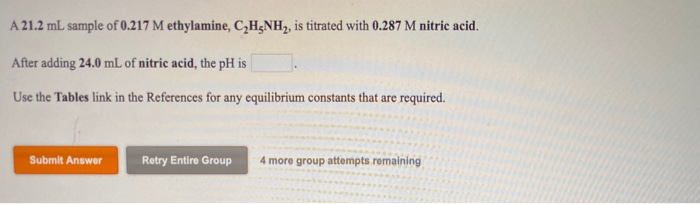A 21.2 ml sample of 0.217 M ethylamine, CH_NH, is titrated with 0.287 M nitric acid. After adding 24.0 mL of nitric acid, the pH is Use the Tables link in the References for any equilibrium constants that are required. Submit Answer Retry Entire Group 4 more group attempts remaining

• ### An aqueous solution of calcium hydroxide is standardized by titration with a 0.190 M solution of...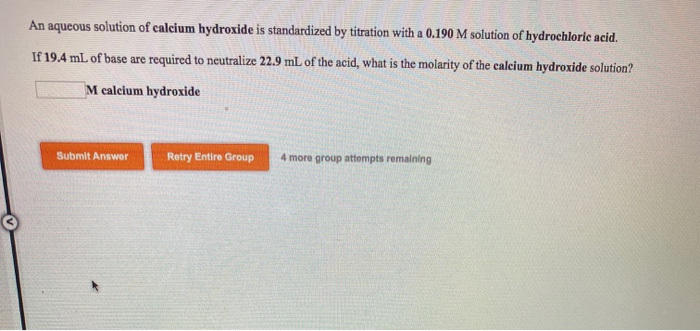An aqueous solution of calcium hydroxide is standardized by titration with a 0.190 M solution of hydrochloric acid. If 19.4 mL of base are required to neutralize 22.9 mL of the acid, what is the molarity of the calcium hydroxide solution? M calcium hydroxide Submit Answer Retry Entire Group 4 more group attempts remaining An aqueous solution of hydrobromic acid is standardized by titration with a 0.155 M solution of calcium hydroxide, If 29.2 mL of base are required to...

• ### A student is asked to standardize a solution of potassium hydroxide. He weighs out 1.10 g...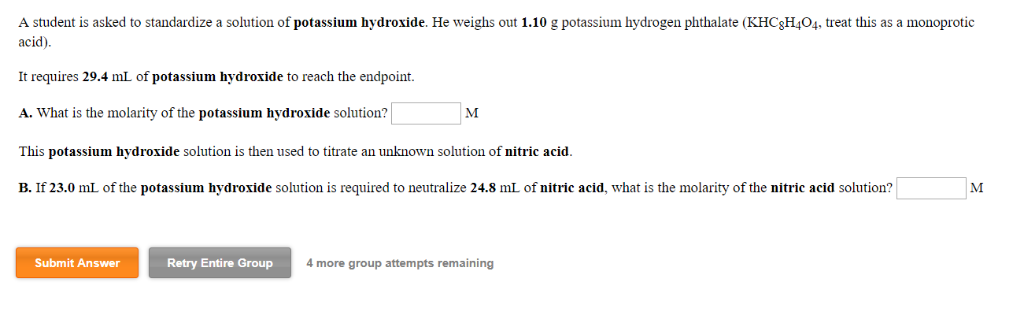A student is asked to standardize a solution of potassium hydroxide. He weighs out 1.10 g potassium hydrogen phthalate (KHCsH404, treat this as a monoprotic acid) It requires 29.4 mL of potassium hydroxide to reach the endpoint. A. What is the molarity of the potassium hydroxide solution? This potassium hydroxide solution is then used to titrate an unknown solution of nitric acid. acid solution?「 1M B. If 23.0 mL of the potassium hydroxide solution is required to neutralize 24.8 ml...